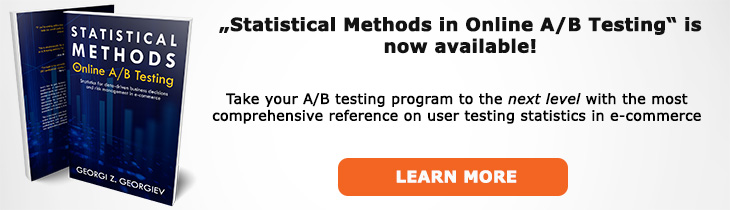# What does "Mean" mean?

Definition of Mean in the context of A/B testing (online controlled experiments).

## What is a Mean?

Aliases: average

The mean, a.k.a. average in A/B testing usually refers to the arithmetic mean of a sample or population. It is calculated as the sum x1, x2, ... , xn divided by n. In an A/B test we compute statistics almost exclusively related to means, with proportions being the mean for a binomial metric which is why we care a lot about knowing or estimating the standard error of the mean (SEM).

Example of means for non-binomial metrics often used in A/B testing are the average order value, the average revenue per user, the average lifetime value, average session duration, average page speed, and so on.

The mean has properties of a good sample statistic since it is an asymptotically consistent estimator, as well as being a finite-sample unbiased estimator, fully-efficient estimator and a sufficient estimator. What this is means, in short, is that as sample size increases the mean consistently approaches the population mean and for any finite number of samples n the mean does not show systematic bias, there is no other estimator that results in faster tests with the same accuracy and there is no other statistic that can be calculated from the same sample that provides any additional information as to the value of the parameter. All of the above properties are transferred to any frequentist hypothesis test or estimation procedure that makes use of the arithmetic mean.

The mean is sensitive to extreme values in the sense that it might shift significantly due to only a few extreme observations. The means is also not guaranteed to coincide with any actual value present in the sample, for example the mean of 1,4, and 10 is 5. The means should not be confused by the median (50% of values are below and above it) or the mode (most common value(s)).

## Related A/B Testing terms

Percent Change

Absolute Difference

Proportion

Standard Error of the Mean

Like this glossary entry? For an in-depth and comprehensive reading on A/B testing stats, check out the book "Statistical Methods in Online A/B Testing" by the author of this glossary, Georgi Georgiev.## Glossary Index by Letter

Select a letter to see all A/B testing terms starting with that letter or visit the Glossary homepage to see all.